# MATLAB 根轨迹图的稳定性分析

MATLAB 根轨迹图的稳定性分析

# MATLAB允许特征方程的根轨迹

1 + G（s）H（s）= 0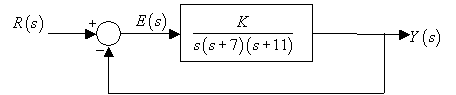1 + G（s）= 0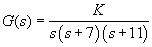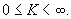s = tf('s');
G = 1/(s*(s+7)*(s+11));
rlocus(G);
axis equal;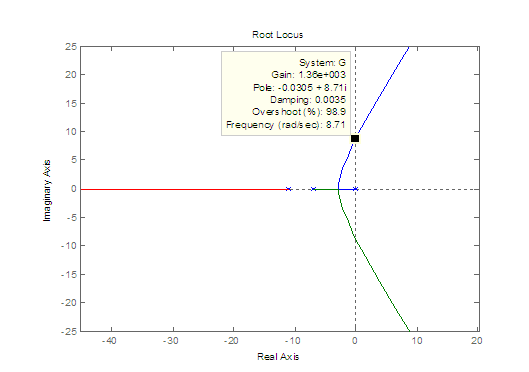>> K = 860;
>> step(feedback(K*G,1),5)
>> hold;
% Current plot held
>> K = 1460;
>> step(feedback(K*G,1),5)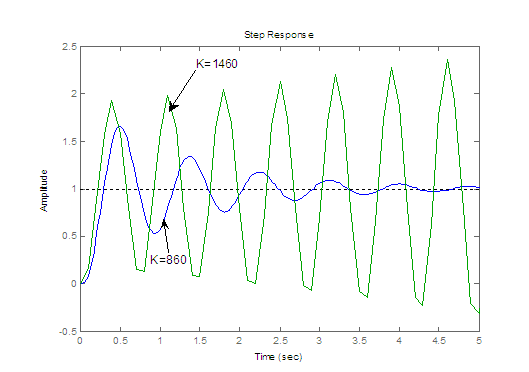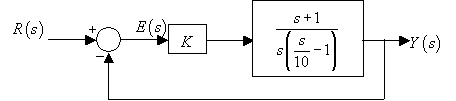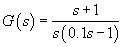clear all;
close all;
s = tf('s');
G = (s+1)/(s*(0.1*s-1));
rlocus(G);
axis equal;
sgrid;
title('Root locus for (s+1)/s(0.1s-1)');
[K,p]=rlocfind(G)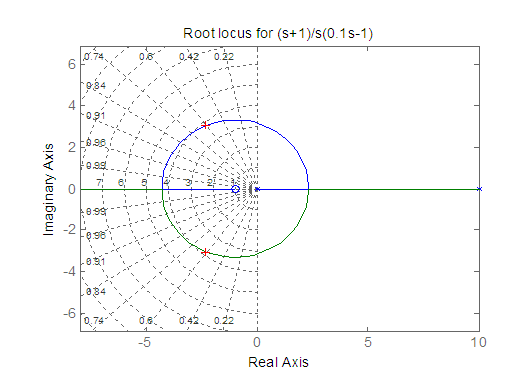selected_point =
-2.2204 + 3.0099i
K =
1.4494
p =
-2.2468 + 3.0734i
-2.2468 - 3.0734i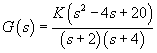s = tf（'s'）;
G =（s ^ 2-4 * s + 20）/（（s + 2）*（s + 4））;
rlocus（G）;
zeta = 0.45;
wn = 0;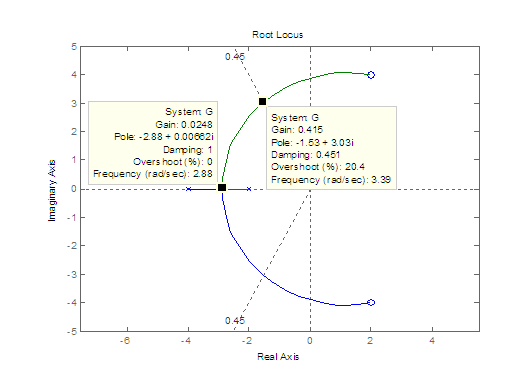03-1112-11
01-114万+
12-275544
03-2497
04-263707
01-17
11-161万+
07-161660
10-081800
10-3078
02-235251
11-12